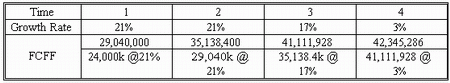### CFA Practice Question

There are 290 practice questions for this topic.

### CFA Practice Question

An analyst is reviewing the following data with respect to York Co.:

• 5 million shares outstanding
• Market risk premium is 6%
• Debt to total capital ratio is 30%
• Risk-free rate is 3%
• York Co beta is 0.92
• Long term debt of \$215 million has an interest cost of 7%
• Tax rate is 40%
• Current free cash flow to the firm (FCFF) is \$24 million
• FCFF is expected to grow 21% over the next two years, 17% in the third year,
• And thereafter, grow at a constant rate of 3%

Which of the following would best estimate the value per share of the firm?
A. \$107.54
B. \$164.25
C. \$138.89
Explanation: Step 1. Compute WACC. WACC = 0.3 x 7% x (1 - 0.4) + 0.7 x 8.5% = 7.2%. Note that 8.5% = 3% + 0.92 x 6%.

Step 2: Forecast future period FCFF:Step 3. Compute the terminal value of the firm at the point that its growth rate stabilizes (since growth stabilizes in year 4, we calculate terminal value as of the end of the year 3):

V3 = FCFF4/(WACC - g) = 42,345,286/(0.072 - 0.03) = 1,008,221.095

Step 4. Compute the PV of all interim FCFFs and the terminal value.

V0 = 29,040,000/1.072 + 35,138,400/1.0722 + 41,111,928/1.0723 + 1,008,221,095/1.0723 = 909,449,376.

Step 5. Compute value of equity.

Equity value = Total value - debt value = 909,449,376 - 215,000,000 = \$694,449,376

Step 6. Computer value per share = \$694,449,376/5,000,000 = 138.89.